# 比特币：一个点对点的电子现金系统算法 同时被 2 个专栏收录49 篇文章 4 订阅

#### 2 交易(Transactions)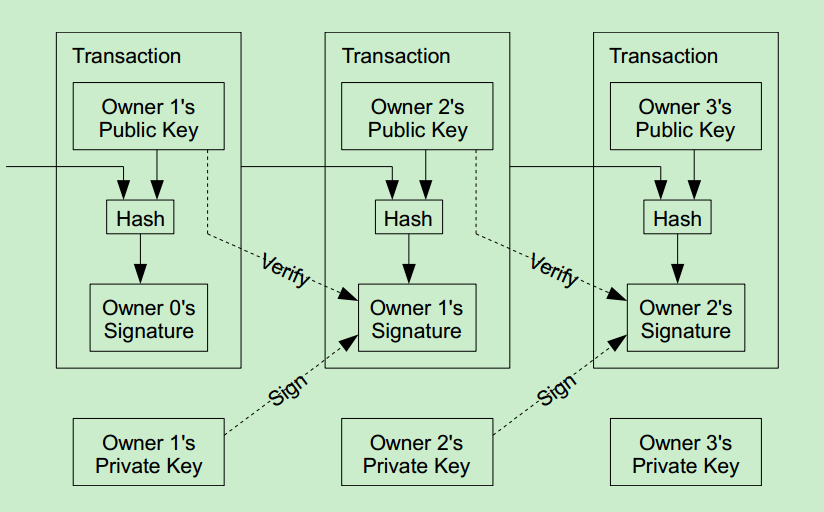#### 3 时间戳服务器(Timestamp Server)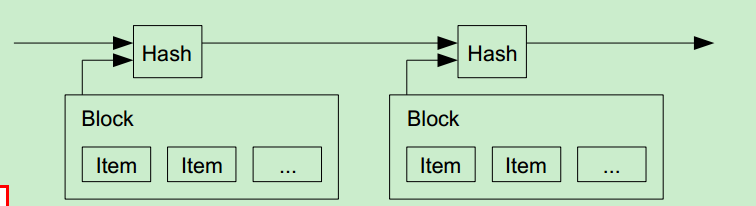#### 4 工作量证明(Proof-of-Work)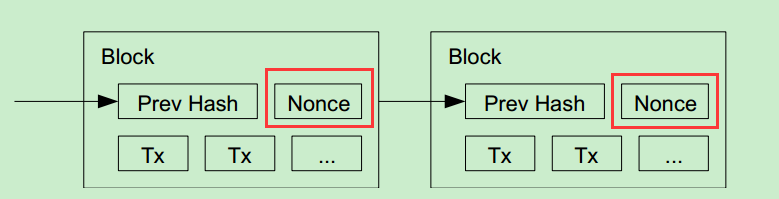#### 5 网络(Network)

• (1) 新的交易向全网进行广播；
• (2) 每一个节点都将收到的交易信息纳入一个区块中；
• (3) 每个节点都尝试在自己的区块中找到一个具有足够难度的工作量证明；
• (4) 当一个节点找到了一个工作量证明，它就向全网进行广播；
• (5) 当且仅当包含在该区块中的所有交易都是有效的且之前未存在过的，其他节点才认同该区块的有效性；
• (6) 其他节点表示他们接受该区块是通过在跟随该区块的末尾，制造新的区块以延长该链条，而将被接受区块的随机散列值视为previous的随机散列值。

#### 7 回收磁盘空间(Reclaiming Disk Space)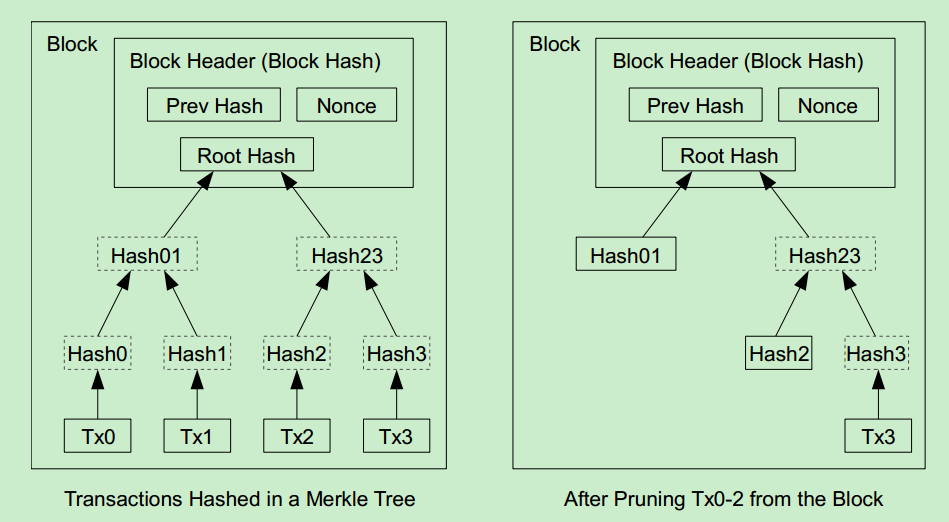#### 8 简化支付确认(Simplified Payment Verification)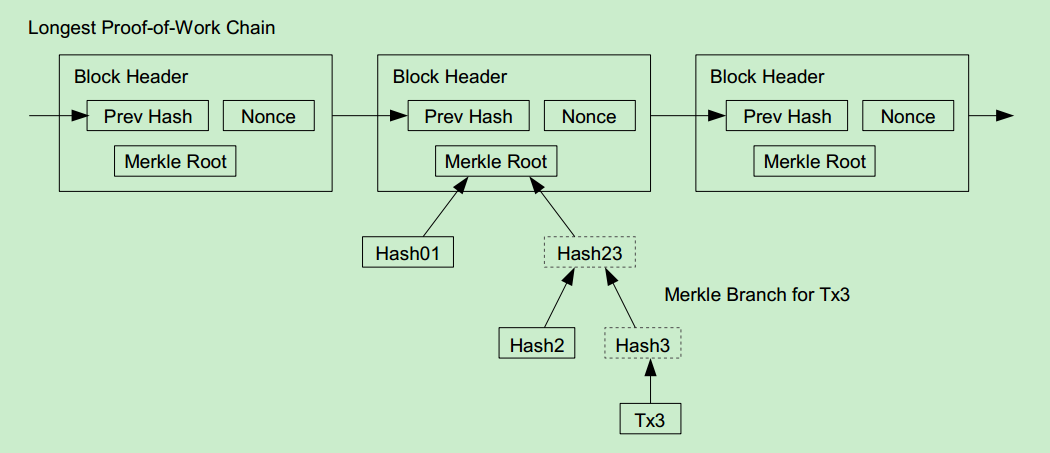#### 9 价值的组合、切分(Combining and Splitting Value)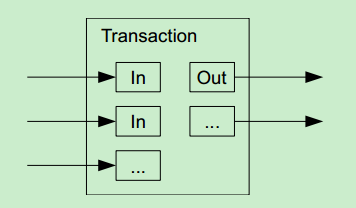#### 10 隐私(Privacy)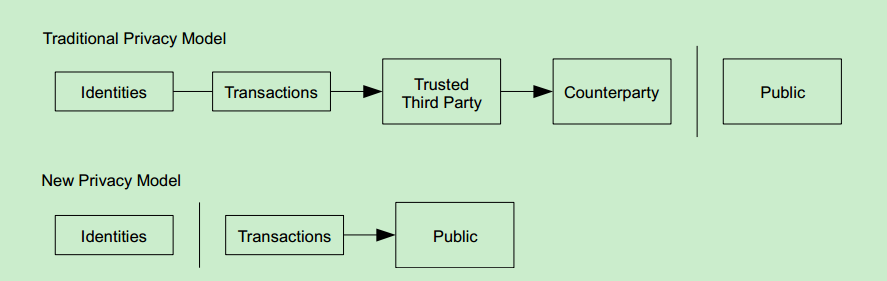#### 11 计算(Calculations)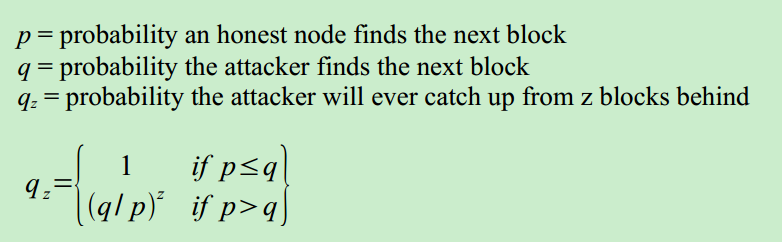λ=zqp λ = z q p

i=0λkeλk!{(qp)zk1k<zk>z} ∑ i = 0 ∞ λ k e − λ k ! { ( q p ) z − k k < z 1 k > z }

1i=0zλkeλk!(1(qp)zk) 1 − ∑ i = 0 z λ k e − λ k ! ( 1 − ( q p ) z − k )

C代码：

#include <math.h>
double AttackerSuccessProbability(double q, int z)
{
double p = 1.0 - q;
double lambda = z * (q / p);
double sum = 1.0;
int i, k;
for (k = 0; k <= z; k++)
{
double poisson = exp(-lambda);
for (i = 1; i <= k; i++)
poisson *= lambda / i;
sum -= poisson * (1 - pow(q / p, z - k));
}
return sum;
}


z=0 P=1.0000000
z=1 P=0.2045873
z=2 P=0.0509779
z=3 P=0.0131722
z=4 P=0.0034552
z=5 P=0.0009137
z=6 P=0.0002428
z=7 P=0.0000647
z=8 P=0.0000173
z=9 P=0.0000046
z=10 P=0.0000012

z=0 P=1.0000000
z=5 P=0.1773523
z=10 P=0.0416605
z=15 P=0.0101008
z=20 P=0.0024804
z=25 P=0.0006132
z=30 P=0.0001522
z=35 P=0.0000379
z=40 P=0.0000095
z=45 P=0.0000024
z=50 P=0.0000006

q=0.10 z=5
q=0.15 z=8
q=0.20 z=11
q=0.25 z=15
q=0.30 z=24
q=0.35 z=41
q=0.40 z=89
q=0.45 z=340

#### 参考文献(References)

 W. Dai, “b-money,” http://www.weidai.com/bmoney.txt, 1998
 H. Massias, X.S. Avila, and J.-J. Quisquater, “Design of a secure timestamping service with minimal
trust requirements,” In 20th Symposium on Information Theory in the Benelux, May 1999.
 S. Haber, W.S. Stornetta, “How to time-stamp a digital document,” In Journal of Cryptology, vol 3, no 2, pages 99-111, 1991.
 D. Bayer, S. Haber, W.S. Stornetta, “Improving the efficiency and reliability of digital time-stamping,” In Sequences II: Methods in Communication, Security and Computer Science, pages 329-334, 1993.
 S. Haber, W.S. Stornetta, “Secure names for bit-strings,” In Proceedings of the 4th ACM Conference on Computer and Communications Security, pages 28-35, April 1997.
 A. Back, “Hashcash - a denial of service counter-measure,”http://www.hashcash.org/papers/hashcash.pdf, 2002.
 R.C. Merkle, “Protocols for public key cryptosystems,” In Proc. 1980 Symposium on Security and Privacy, IEEE Computer Society, pages 122-133, April 1980.
 W. Feller, “An introduction to probability theory and its applications,” 1957.

09-04
06-12512212-142631
08-143451
10-22
11-154649
11-042263
03-26763
06-251259
12-231681
12-24766
©️2021 CSDN 皮肤主题: Age of Ai 设计师:meimeiellie点击重新获取扫码支付1.余额是钱包充值的虚拟货币，按照1:1的比例进行支付金额的抵扣。
2.余额无法直接购买下载，可以购买VIP、C币套餐、付费专栏及课程。余额充值